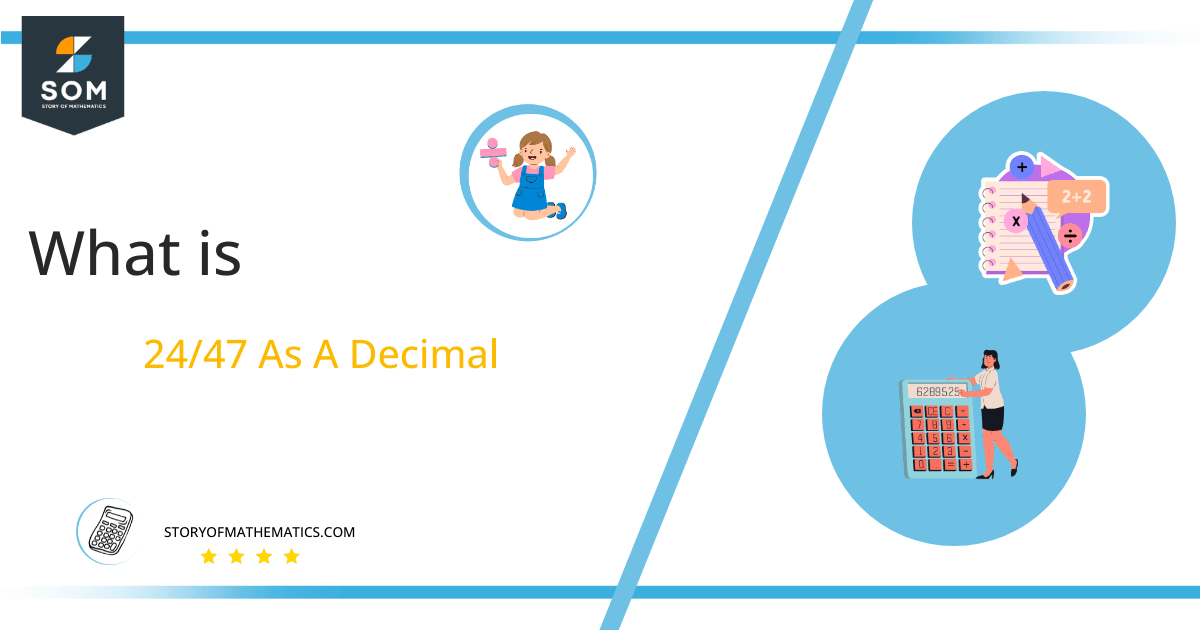# What Is 24/47 as a Decimal + Solution With Free Steps

The fraction 24/47 as a decimal is equal to 0.510.

The corresponding decimal value of a Fraction is obtained, when its two components are divided. Depending on the values of the fraction’s components, the resulting decimal value can either be terminating or non-recurring, or it can be recurring or non-terminating.

Here, we are more interested in the division types that result in a Decimal value, as this can be expressed as a Fraction. We see fractions as a way of showing two numbers having the operation of Division between them that result in a value that lies between two Integers.Now, we introduce the method used to solve said fraction to decimal conversion, called Long Division, which we will discuss in detail moving forward. So, let’s go through the Solution of fraction 24/47.

## Solution

First, we convert the fraction components, i.e., the numerator and the denominator, and transform them into the division constituents, i.e., the Dividend and the Divisor, respectively.

This can be done as follows:

Dividend = 24

Divisor = 47

Now, we introduce the most important quantity in our division process: the Quotient. The value represents the Solution to our division and can be expressed as having the following relationship with the Division constituents:

Quotient = Dividend $\div$ Divisor = 24 $\div$ 47

This is when we go through the Long Division solution to our problem, as shown in figure 1.Figure 1

## 24/47 Long Division Method

We start solving a problem using the Long Division Method by first taking apart the division’s components and comparing them. As we have 24 and 47, we can see how 24 is Smaller than 47, and to solve this division, we require that 24 be Bigger than 47.

This is done by multiplying the dividend by 10 and checking whether it is bigger than the divisor or not. If so, we calculate the Multiple of the divisor closest to the dividend and subtract it from the Dividend. This produces the Remainder, which we then use as the dividend later.

Now, we begin solving for our dividend 24, which after getting multiplied by 10 becomes 240.

We take this 240 and divide it by 47; this can be done as follows:

240 $\div$ 47 $\approx$ 5

Where:

47 x 5 = 35

This will lead to the generation of a Remainder equal to 240 – 235 = 5. Now this means we have to repeat the process by Converting the 5 into 50 and solving for that:

50 $\div$ 47 $\approx$ 1

Where:

47 x 1 = 47

This, therefore, produces another Remainder which is equal to 50 – 47 = 3. Now we must solve this problem to Third Decimal Place for accuracy, so we repeat the process with dividend 30.

30 $\div$ 47 $\approx$ 0

Where:

47 x 0 = 0

Finally, we have a Quotient generated after combining the three pieces of it as 0.510=z, with a Remainder equal to 30.Images/mathematical drawings are created with GeoGebra.